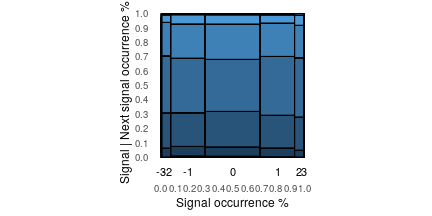This R package offers novel time series visualisations. It is based on `ggplot2` and offers `geom`s and pre-packaged functions for easily creating any of the offered charts. Some examples are listed below.

This package can be installed from github by installing `devtools` library and then running the following command - `devtools::install_github('Ather-Energy/ggTimeSeries')`.

## Line Charts Legacy

IoT devices generate a lot of sequential data over time, also called time series data. Legacy portrayals of such data would centre around line charts. Line charts have reportedly been around since the early 1700s (source: Wikipedia) and we have nothing against them. They facilitate trend detection and comparison, are simple to draw, and easy to understand; all in all a very well behaved visualisation. In modern times, their use is widespread from the heartbeat monitor at a hospital to the multiple-monitor display at a trader's desk.

``````#>  "Excel 97 look recreated in R with the ggthemes package"
``````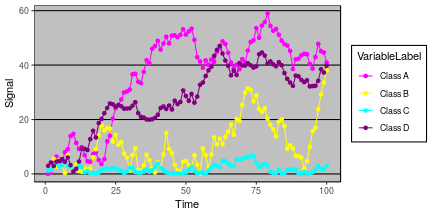## Alternatives

However there are cases when the data scientist becomes more demanding and specific. Five alternatives available to such a data scientist are listed below. All of these options are available as `geom`s or packaged functions in the `ggplot2` based `ggTimeSeries` package.

Before that, setting a minimal theme -

``````minimalTheme = theme_set(theme_bw(12))
minimalTheme = theme_update(
axis.ticks = element_blank(),
legend.position = 'none',
strip.background = element_blank(),
panel.border = element_blank(),
panel.background = element_blank(),
panel.grid = element_blank()
)
``````

### Calendar Heatmap

Available as `stat_calendar_heatmap` and `ggplot_calendar_heatmap`.

A calendar heatmap is a great way to visualise daily data. Its structure makes it easy to detect weekly, monthly, or seasonal patterns.

``````
# creating some data
set.seed(1)
dtData = data.table(
DateCol = seq(
as.Date("1/01/2014", "%d/%m/%Y"),
as.Date("31/12/2015", "%d/%m/%Y"),
"days"
),
ValueCol = runif(730)
)
dtData[, ValueCol := ValueCol + (strftime(DateCol,"%u") %in% c(6,7) * runif(1) * 0.75), .I]
dtData[, ValueCol := ValueCol + (abs(as.numeric(strftime(DateCol,"%m")) - 6.5)) * runif(1) * 0.75, .I]

# base plot
p1 = ggplot_calendar_heatmap(
dtData,
'DateCol',
'ValueCol'
)

p1 +
xlab(NULL) +
ylab(NULL) +
scale_fill_continuous(low = 'green', high = 'red') +
facet_wrap(~Year, ncol = 1)
``````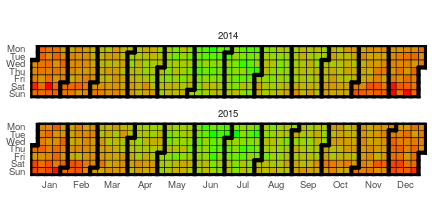``````

# creating some categorical data
dtData[, CategCol := letters[1 + round(ValueCol * 7)]]

# base plot
p2 = ggplot_calendar_heatmap(
dtData,
'DateCol',
'CategCol'
)

p2 +
xlab(NULL) +
ylab(NULL) +
facet_wrap(~Year, ncol = 1)
``````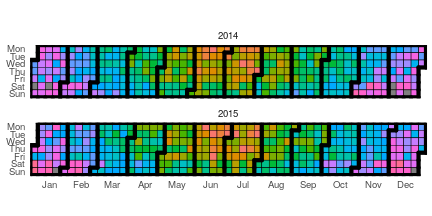### Horizon Plots

Available as `stat_horizon` and `ggplot_horizon`.

Imagine an area chart which has been chopped into multiple chunks of equal height. If you overlay these chunks one on top of the the other, and colour them to indicate which chunk it is, you get a horizon plot. Horizon plots are useful when visualising y values spanning a vast range but with a skewed distribution, and / or trying to highlight outliers without losing context of variation in the rest of the data.

``````
# creating some data
set.seed(1)
dfData = data.frame(x = 1:1000, y = cumsum(rnorm(1000)))

# base plot
p1 = ggplot_horizon(dfData, 'x', 'y')

print("If you're seeing any vertical white stripes, it's a display thing.")
#>  "If you're seeing any vertical white stripes, it's a display thing."
p1 +
xlab(NULL) +
ylab(NULL) +
scale_fill_continuous(low = 'green', high = 'red') +
coord_fixed( 0.5 * diff(range(dfData\$x)) / diff(range(dfData\$y)))
``````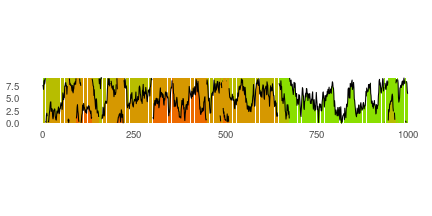### Steamgraphs

Available as `stat_steamgraph`.

A steamgraph is a more aesthetically appealing version of a stacked area chart. It tries to highlight the changes in the data by placing the groups with the most variance on the edges, and the groups with the least variance towards the centre. This feature in conjunction with the centred alignment of each of the contributing areas makes it easier for the viewer to compare the contribution of any of the components across time.

``````# creating some data
set.seed(10)
dfData = data.frame(
Time = 1:1000,
Signal = abs(
c(
cumsum(rnorm(1000, 0, 3)),
cumsum(rnorm(1000, 0, 4)),
cumsum(rnorm(1000, 0, 1)),
cumsum(rnorm(1000, 0, 2))
)
),
VariableLabel = c(rep('Class A', 1000), rep('Class B', 1000), rep('Class C', 1000), rep('Class D', 1000))
)

# base plot
p1 = ggplot(dfData, aes(x = Time, y = Signal, group = VariableLabel, fill = VariableLabel)) +
stat_steamgraph()

p1 +
xlab(NULL) +
ylab(NULL) +
coord_fixed( 0.2 * diff(range(dfData\$Time)) / diff(range(dfData\$Signal)))
``````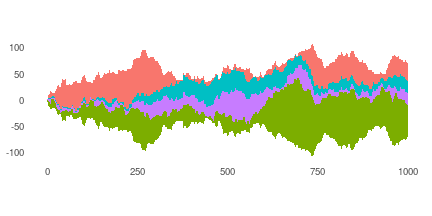### Waterfall

Available as `stat_waterfall` and `ggplot_waterfall`.

Rather than the values itself, a waterfall plot tries to bring out the changes in the values.

``````# creating some data
set.seed(1)
dfData = data.frame(x = 1:100, y = cumsum(rnorm(100)))

# base plot
p1 = ggplot_waterfall(
dtData = dfData,
'x',
'y'
)

p1 +
xlab(NULL) +
ylab(NULL)
``````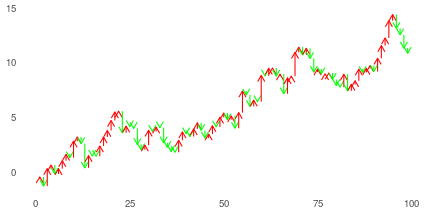### Occurrence Dot Plot

Available as `stat_occurrence`.

This one is a favourite in infographics. For rare events, the reader would find it convenient to have the count of events encoded in the chart itself instead of having to map the value back to the Y axis.

``````# creating some data
set.seed(1)
dfData = data.table(x = 1:100, y = floor(4 * abs(rnorm(100, 0 , 0.4))))

# base plot
p1 = ggplot(dfData, aes(x =x, y = y) )+
stat_occurrence()

p1 +
xlab(NULL) +
ylab(NULL) +
coord_fixed(ylim = c(0,1 + max(dfData\$y)))
``````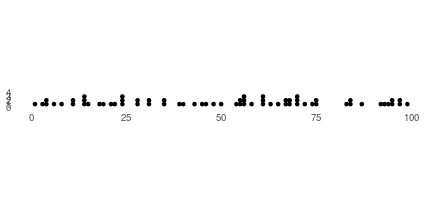### Marimekko or Mosaik

Available as `stat_marimekko`.

A marimekko plot, or a mosaic plot, visualises the co-occurrence of two categorical / ordinal variables. In case of a time series, it could be used to visualise the proportion of transitions from one state to another by considering each state to be a category and plotting the occurrence of current category vs. next category. The span on the horizontal represents the overall occurrence of the xbucket argument. The span on the vertical represents the marginal occurrence of the ybucket argument when xbucket was the previous state. The area of a block indicates the proportion of occurrence of that pair in the entire dataset.

``````# creating some data
set.seed(1)

dfData = data.frame(Signal = pmax(pmin(rnorm(10000), 3), -3))

dfData2 = data.frame(
NextSignal = round(tail(dfData\$Signal, -1),0),
Weight = 1
)

# base plot
p1 = ggplot(dfData2, aes(xbucket = Signal, ybucket = NextSignal, fill = NextSignal, weight = Weight) )+
stat_marimekko(color = 'black', xlabelyposition = -0.1)